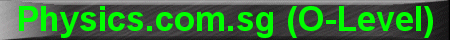(New tips are continually added to these pages.  Check back in a few months' time for more)

TOPIC 15:    Electric Circuits

Tip 1:

To find the total resistance of several resistors connected in series, simply add up their resistances.  This is pretty straight forward, as follows:

RT = R1 + R2 + R3

For resistances in parallel, some short-cut formulae exist which can save both time and effort.

We know that for resistances in parallel, let's say R1, R2 and R3, then:

1/RT = 1/R1 + 1/R2 + 1/R3

If the 3 resistors have the same value, eg. R, then:

1/RT = 1/R + 1/R + 1/R = 3/R

Hence, RT = R/3

In general, if there are N number of resistors R in parallel, their combined resistance is R/N

Another short-cut:

If two resistors R1 and R2 are in parallel, then:

1/RT = 1/R1 + 1/R2

1/RT = (R1 + R2)/(R1R2)

RT = (R1R2)/(R1 + R2)

ie. their combined resistance is equal to their product divided by their sum.

eg. resistors 3W and 6W are in parallel, their combined resistance is:

(3 x 6)/(3 + 6) = 18/9 = 2 W